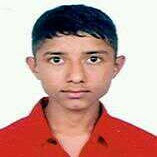# Syllabus - Engineering Mathematics I - for TU IOE all BE first year first semester

ENGINEERING MATHEMATICS I
SH 401

Lecture:  3                                                                                                                  Year: I
Tutorial: 2                                                                                                                  Part: I
Practical :
Course Objectives:    To provide students a sound knowledge of calculus and
analytic geometry to apply them in their relevant fields.

1.          Derivatives and their Applications                                                                                                                  (14 hours)
1.1.       Introduction
1.2.       Higher order derivatives
1.3.       Mean value theorem
1.3.1.     Rolle’s Theorem
1.3.2.     Lagrange’s mean value theorem
1.3.3.     Cauchy’s mean value theorem
1.4.       Power series of single valued function
1.4.1.     Taylor’s series
1.4.2.     Maclaurin’s series
1.5.       Indeterminate forms; L’Hospital rule
1.6.       Asymptotes to Cartesian and polar curves
1.7.       Pedal equations to Cartesian and polar curves; curvature and radius of curvature

2.          Integration and its Applications                                                                                                                       (11 hours)
2.1.       Introduction
2.2.       Definite integrals and their properties
2.3.       Improper integrals
2.4.       Differentiation under integral sign
2.5.       Reduction formula; Beta Gama functions
2.6.       Application of integrals for finding areas, arc length, surface and
solid of revolution in the plane for Cartesian and polar curves

3.          Plane Analytic Geometry                                                                                                                                      (8 hours)
3.1.       Transformation of coordinates: Translation and rotation
3.2.       Ellipse and hyperbola; Standard forms, tangent, and normal
3.3.       General equation of conics in Cartesian and polar forms

4.          Ordinary Differential Equations and their Applications                                                                          (12 hours)
4.1.       First order and first degree differential equations
4.2.       Homogenous differential equations
4.3.       Linear differential equations
4.4.       Equations reducible to linear differential equations; Bernoulli’s equation
4.5.       First order and higher degree differential equation; Clairaut’s equation
4.6.       Second order and first degree linear differential equations with constant          coefficients.
4.7.       Second order and first degree linear differential equations with variable coefficients; Cauchy’s equations
4.8.       Applications in engineering field

Reference books:
1.       Erwin Kreyszig, Advance Engineering Mathematics , John Wiley and Sons Inc
2.       Thomas,Finney,Calculus and Analytical geometry Addison- Wesley
3.       M. B. Singh, B. C. Bajrachrya,  Differential calculus, Sukunda  Pustak Bhandar,Nepal
4.       M. B. Singh,  S. P. Shrestha, Applied Mathematics,
5.       G.D. Pant, G. S. Shrestha, Integral Calculus and Differential Equations, Sunila Prakashan,Nepal
6.       M. R. Joshi, Analytical Geometry, SukundaPustak Bhandar,Nepal
7.       S. P. Shrestha, H. D. Chaudhary, P. R. Pokharel,
A Textbook of Engineering Mathematics - Vol I
8.       Santosh Man Maskey, Calculus, Ratna Pustak Bhandar, Nepal

Evaluation Scheme

The questions will cover all the chapters in the syllabus. The evaluation scheme will be as indicated in the table below:

 Chapters Hours Mark distribution* 1. 14 25 2. 11 20 3. 08 15 4. 12 20 Total 45 80

* There may be minor deviation in marks distribution.
###### Share To: